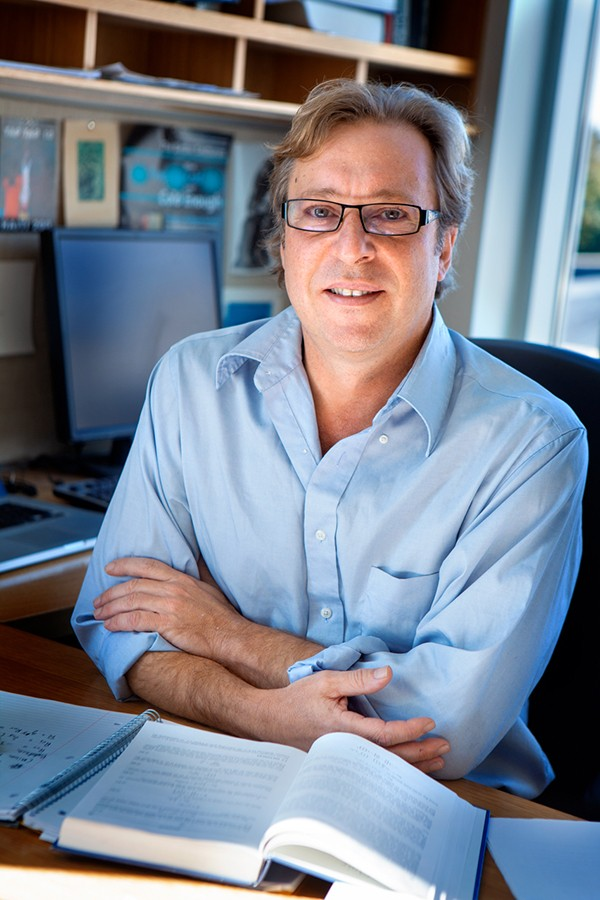Skip to main content

# Physicist offers new take on million-dollar math problem

The Riemann hypothesis – an unsolved problem in pure mathematics, the solution of which would have major implications in number theory and encryption – is one of the seven \$1 million Millennium Prize Problems.First proposed by Bernhard Riemann in 1859, the hypothesis relates to the distribution of prime numbers.

Cornell mathematical physicist André LeClair – in research published in February in the Journal of Statistical Mechanics: Theory and Experiment – offers a possible path to a solution, rather than a straight proof.

“We make one assumption, a fully plausible conjecture. From this one assumption, we show that a lot of things would follow,” said LeClair, a professor in the College of Arts and Sciences. “We don’t have a 100% rigorous proof, but rather a very promising strategy with connections to physics. Even if we can’t fully prove our main assumption, we’ve shed light on where to look.”

Before the 20th century, mathematics and physics were more unified, but divided more recently because of the explosion of scientific knowledge, according to mathematician Steve Gonek of the University of Rochester. Riemann himself studied physical problems as well as “pure” mathematical ones.

“Nevertheless, even today both subjects share many of the same tools and points of view. In fact, there are countless examples of mathematical physicists contributing wonderful insights into mathematical questions, and pure mathematicians helping to understand deep physical questions,” Gonek said. “While categorization into subject areas is useful, we should not be fooled by it: Knowledge transcends categories.”

LeClair has delivered talks on his approach at the Riemann Center in Hannover, Germany; at the Isaac Newton Center for Mathematical Sciences in Cambridge, England; at Ecole Normal Superieur in Paris; and at Stanford University. He has published four papers on the topic.

The Millennium Prize Problems are a contest run by the Clay Mathematics Institute. In May 2000, the institute identified seven longstanding math problems; a correct solution to any of the problems will result in a \$1 million prize.

Linda Glaser is a staff writer for the College of Arts and Sciences.

## Contact

Cornell Chronicle
312 College Ave
Ithaca, NY 14850
607-255-4206
cunews@cornell.edu